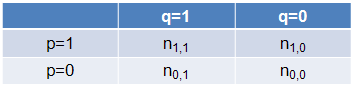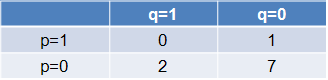# 1(b).2.1: Measures of Similarity and DissimilarityPrinter-friendly version

### Similarity and Dissimilarity

Distance or similarity measures are essential to solve many pattern recognition problems such as classification and clustering. Various distance/similarity measures are available in literature to compare two data distributions.  As the names suggest, a similarity measures how close two distributions are. For multivariate data complex summary methods are developed to answer this question.

Similarity Measure

• Numerical measure of how alike two data objects are.
• Often falls between 0 (no similarity) and 1 (complete similarity).

Dissimilarity Measure

• Numerical measure of how different two data objects are.
• Range from 0 (objects are alike) to ∞ (objects are different).

Proximity refers to a similarity or dissimilarity.

#### Similarity/Dissimilarity for Simple Attributes

Here, p and q are the attribute values for two data objects.

 Attribute Type Similarity Dissimilarity Nominal $s=\begin{cases}1 & \text{ if } p=q \\ 0 & \text{ if } p\neq q\end{cases}$ $d=\begin{cases}0 & \text{ if } p=q \\ 1 & \text{ if } p\neq q\end{cases}$ Ordinal $s=1-\frac{\left \| p-q \right \|}{n-1}$ (values mapped to integer 0 to n-1, where n is the number of values) $d=\frac{\left \| p-q \right \|}{n-1}$ Interval or Ratio $s=1-\left \| p-q \right \|, s=\frac{1}{1+\left \| p-q \right \|}$ $d=\left \| p-q \right \|$

### Common Properties of Dissimilarity Measures

Distance, such as the Euclidean distance, is a dissimilarity measure and has some well known properties:

1. d(p, q) ≥ 0 for all p and q, and d(p, q) = 0 if and only if p = q,
2. d(p, q) = d(q,p) for all p and q,
3. d(p, r) ≤ d(p, q) + d(q, r) for all p, q, and r, where d(p, q) is the distance (dissimilarity) between points (data objects), p and q.

A distance that satisfies these properties is called a metric.Following is a list of several common distance measures to compare multivariate data. We will assume that the attributes are all continuous.

#### Euclidean Distance

Assume that we have measurements xiki = 1, … , N, on variables k = 1, … , p (also called attributes).

The Euclidean distance between the ith and jth objects is

$d_E(i, j)=\left(\sum_{k=1}^{p}\left(x_{ik}-x_{jk} \right) ^2\right)^\frac{1}{2}$

for every pair (i, j) of observations.

The weighted Euclidean distance is

$d_{WE}(i, j)=\left(\sum_{k=1}^{p}W_k\left(x_{ik}-x_{jk} \right) ^2\right)^\frac{1}{2}$

If scales of the attributes differ substantially, standardization is necessary.

#### Minkowski Distance

The Minkowski distance is a generalization of the Euclidean distance.

With the measurement,  xiki = 1, … , N,  k = 1, … , p, the Minkowski distance is

$d_M(i, j)=\left(\sum_{k=1}^{p}\left | x_{ik}-x_{jk} \right | ^ \lambda \right)^\frac{1}{\lambda},$

where λ ≥ 1.  It is also called the Lλ metric.

• λ = 1 : L1 metric, Manhattan or City-block distance.
• λ = 2 : L2 metric, Euclidean distance.
• λ → ∞ : L metric, Supremum distance.

$\lim{\lambda \to \infty}=\left( \sum_{k=1}^{p}\left | x_{ik}-x_{jk} \right | ^ \lambda \right) ^\frac{1}{\lambda} =\text{max}\left( \left | x_{i1}-x_{j1}\right| , ... , \left | x_{ip}-x_{jp}\right| \right)$

Note that λ and p are two different parameters. Dimension of the data matrix remains finite.

#### Mahalanobis Distance

Let X be a N × p matrix. Then the ith row of X is

$x_{i}^{T}=\left( x_{i1}, ... , x_{ip} \right)$

The Mahalanobis distance is

$d_{MH}(i, j)=\left( \left( x_i - x_j\right)^T \Sigma^{-1} \left( x_i - x_j\right)\right)^\frac{1}{2}$

where ∑ is the p×p sample covariance matrix.

### Self-check

Calculate the answers to these questions by yourself and then click the icon on the left to reveal the answer.

1. We have   $X= \begin{pmatrix} 1 & 3 & 1 & 2 & 4\\ 1 & 2 & 1 & 2 & 1\\ 2 & 2 & 2 & 2 & 2 \end{pmatrix}$.

• Calculate the Euclidan distances.
• Calculate the Minkowski distances (λ=1 and λ→∞ cases).

2. We have   $X= \begin{pmatrix} 2 & 3 \\ 10 & 7 \\ 3 & 2 \end{pmatrix}$.

• Calculate the Minkowski distance (λ = 1, λ = 2, and λ → ∞ cases) between the first and second objects.
• Calculate the Mahalanobis distance between the first and second objects.

### Common Properties of Similarity Measures

Similarities have some well known properties:

1. s(p, q) = 1 (or maximum similarity) only if p = q,
2. s(p, q) = s(q, p) for all p and q, where s(p, q) is the similarity between data objects, p and q.

#### Similarity Between Two Binary Variables

The above similarity or distance measures are appropriate for continuous variables. However, for binary variables a different approach is necessary.Simple Matching and Jaccard Coefficients

• Simple matching coefficient = (n1,1+ n0,0) / (n1,1 + n1,0 + n0,1 + n0,0).
• Jaccard coefficient =  n1,1 / (n1,1 + n1,0 + n0,1).

### Self-check

The frequency table is